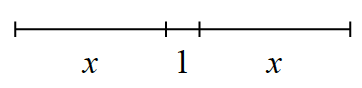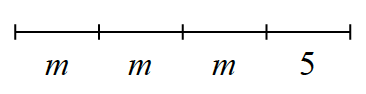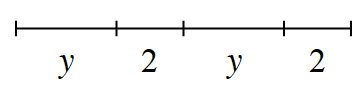### Home > CC1 > Chapter Ch4 > Lesson 4.2.4 > Problem4-82

4-82.

Use an algebraic expression to represent each sequence of lengths shown below. Homework Help ✎

1.Think of this as being a tightrope for Croakie to cross. How would you describe his path using this picture?

2x + 1 or x + 1 + x

2.This problem is very similar to part (a).
If the lengths labeled ''m'' were instead labeled as ''x'', how would you write the expression?

3m + 5 or m + m + m + 5

3.Use parts (a) and (b) as guides if you are having trouble. Remember that the variable represents a number, so you can use it in an expression just as you would a number.